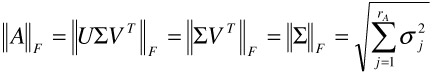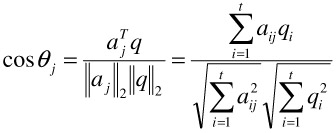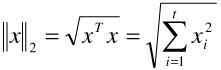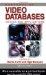# 4. Latent Semantic Indexing

## 4. Latent Semantic Indexing

Latent Semantic Indexing (LSI) was introduced to overcome a fundamental problem that plagues existing textual retrieval techniques. The problem is that users want to retrieve documents on the basis of conceptual content, while individual keywords provide unreliable evidence about the conceptual meaning of a document. There are usually many ways to express a given concept. Therefore, the literal terms used in a user query may not match those of a relevant document. In addition, most words have multiple meanings and are used in different contexts. Hence, the terms in a user query may literally match the terms in documents that are not of any interest to the user at all.

In information retrieval these two problems are addressed as synonymy and polysemy. The concept synonymy is used to describe the fact that there are many ways to refer to the same object. Users in different contexts, or with different needs, knowledge, or linguistic habits will describe the same concept using different terms. The prevalence of synonyms tends to decrease the recall performance of the retrieval. By polysemy we refer to the fact that most words have more than one distinct meaning. In different contexts or when used by different people, the same term takes on a varying referential significance. Thus, the use of a term in a query may not necessarily mean that a document containing the same term is relevant at all. Polysemy is one factor underlying poor precision performance of the retrieval .

Latent semantic indexing tries to overcome the deficiencies of term-matching retrieval. It is assumed that there exists some underlying latent semantic structure in the data that is partially obscured by the randomness of word choice. Statistical techniques are used to estimate this latent semantic structure, and to get rid of the obscuring noise.

The LSI technique makes use of the Singular Value Decomposition (SVD). We take a large matrix of term-document association and construct a semantic space wherein terms and documents that are closely associated are placed near to each other. The singular value decomposition allows the arrangement of the space to reflect the major associative patterns in the data, and ignore the smaller, less important influences. As a result, terms that did not actually appear in a document may still end up close to the document, if that is consistent with the major patterns of association in the data. Position in the transformed space then serves as a new kind of semantic indexing. Retrieval proceeds by using the terms in a query to identify a point in the semantic space, and documents in its neighborhood are returned as relevant results to the query.

Latent semantic indexing is based on the fact that the term-document association can be formulated by using the vector space model, in which each document is represented as a vector, where each vector component reflects the importance of a particular term in representing the semantics of that document. The vectors for all the documents in a database are stored as the columns of a single matrix. Latent semantic indexing is a variant of the vector space model in which a low-rank approximation to the vector space representation of the database is employed. That is, we replace the original matrix by another matrix that is as close as possible to the original matrix but whose column space is only a subspace of the column space of the original matrix. Reducing the rank of the matrix is a means of removing extraneous information or noise from the database it represents. According to , latent semantic indexing has achieved average or above average performance in several experiments with the TREC collections.

In the vector space model, a vector is used to represent each item or document in a collection. Each component of the vector reflects a particular keyword associated with the given document. The value assigned to that component reflects the importance of the term in representing the semantics of the document.

A database containing a total of d documents described by t terms is represented as a t d term-document matrix A. The d vectors representing the d documents form the columns of the matrix. Thus, the matrix element ay is the weighted frequency at which term i occurs in document j. The columns of A are called the document vectors, and the rows of A are the term vectors. The semantic content of the database is contained in the column space of A, meaning that the document vectors span that content. We can exploit geometric relationships between document vectors to model similarity and differences in content. Meanwhile, we can also compare term vectors geometrically in order to identify similarity and differences in term usage.

A variety of schemes are available for weighting the matrix elements. The element aij of the term-document matrix A is often assigned such values as aij = lijgi. The factor gi is called the global weight, reflecting the overall value of term i as an indexing term for the entire collection. Global weighting schemes range from simple normalization to advanced statistics-based approaches . The factor lij is a local weight that reflects the importance of term i within document j itself. Local weights range in complexity from simple binary values to functions involving logarithms of term frequencies. The latter functions have a smoothing effect in that high-frequency terms having limited discriminatory value are assigned low weights.

The Singular Value Decomposition (SVD) is a dimension reduction technique which gives us reduced-rank approximations to both the column space and row space of the vector space model. SVD also allows us to find a rank-k approximation to a matrix A with minimal change to that matrix for a given value of k . The decomposition is defined as follows,

A = UVT

where U is the t t orthogonal matrix having the left singular vectors of A as its columns, V is the d x d orthogonal matrix having the right singular vectors of A as its columns, and is the t d diagonal matrix having the singular values σ1 σ2 _ σr of the matrix A in order along its diagonal, where r min(t, d). This decomposition exists for any given matrix A .

The rank rA of the matrix A is equal to the number of nonzero singular values. It follows directly from the orthogonal invariance of the Frobenius norm that || A ||F is defined in terms of those values,The first rA columns of matrix U are a basis for the column space of matrix A, while the first rA rows of matrix VT are a basis for the row space of matrix A. To create a rank-k approximation Ak to the matrix A, where k rA, we can set all but the k largest singular values of A to be zero. A classic theorem about the singular value decomposition by Eckart and Young  states that the distance between the original matrix A and its rank-k approximation is minimized by the approximation Ak. The theorem further shows how the norm of that distance is related to singular values of matrix A. It is described asHere Ak = Uk k VkT , where Uk is the t k matrix whose columns are the first k columns of matrix U, Vk is the d k matrix whose columns are the first k columns of matrix V, and k is the k k diagonal matrix whose diagonal elements are the k largest singular values of matrix A. Using the SVD to find the approximation Ak guarantees that the approximation is the best that can be achieved for any given choice of k.

In the vector space model, a user queries the database to find relevant documents, using the vector space representation of those documents. The query is also a set of terms, with or without weights, represented by using a vector just like the documents. The matching process is to find the documents most similar to the query in the use and weighting of terms. In the vector space model, the documents selected are those geometrically closest to the query in the transformed semantic space.

One common measure of similarity is the cosine of the angle between the query and document vectors. If the term-document matrix A has columns aj, j = 1, 2, .,. d, those d cosines are computed according to the following formulafor j = 1, 2, .,. d, where the Euclidean vector norm ||x||2 is defined byfor any t-dimensional vector x.

The latent semantic indexing technique has been successfully applied to textual information retrieval, in which it shows distinctive power of finding the latent correlation between terms and documents [2, 3, 7]. This inspired us to apply LSI to content-based image retrieval. In a previous study [38, 39], we made use of the power of LSI to reveal the underlying semantic nature of image contents, and thus to find the correlation between image features and the semantics of the image or its objects. Then in  we further extended the power of LSI to the domain of Web document retrieval by applying it to both textual and visual contents of Web documents and the experimental results verified that latent semantic indexing helps improve the retrieval performance by uncovering the semantic structure of Web documents.Handbook of Video Databases: Design and Applications (Internet and Communications)
ISBN: 084937006X
EAN: 2147483647
Year: 2003
Pages: 393